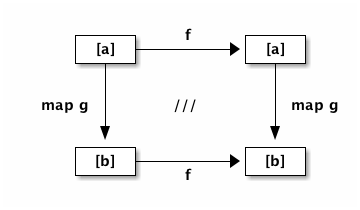Let’s start our Frege journey in a rather unconventional way.

Instead of showing the syntax for `"Hello, World"`, we begin with an example of equational reasoning.

Frege brings this novel kind of designing and re-factoring solutions to the JVM. Its way of purely functional programming bases our programs on a solid foundation that roots deeply in the fabric of mathematics.

So while in Frege you can of course do everything that you can do in any other language, we start with a Frege benefit that you will not get in any other JVM language.

Enjoy the show!

## A Magical Trick

First, think of a function f that maps a lists of arbitrary element types to another list of the exact same type.

In Frege terms, such a function f is declared as

``f :: [a] -> [a]``
 Note Assume nothing We cannot assume anything about the features of a. That very much limits what f can possibly do. For example, f cannot add "plus one" to all elements since a is not known to support arithmetic.

Now, really, go for it. Think of any such function f and keep it secret!

Second, think of any specific function g with type `a → b` that for a given type returns a value of some other type. For example, you can choose:

An example for g as a function from Int to String
``````g :: Int -> String
g x = show x ++ show x ++ show x``````

That is, `g 1` would return the String `"111"`.

But you can really think of any specific function g you fancy.

Here is the first part of the trick
• make a list of values that could be passed into g (a list of Int values in our example above)

• now, apply your secret f to that list

• then feed the result into `map g`

• you now have a list of return values of g

Do you have it?

Now the second part
• start with the same list as you started part 1 with

• now, first apply `map g` to the list

• then apply your secret function f

 Important TADAAA! You will arrive at the same result again!

Let’s try with an example. Assume we had chosen the `reverse` function for f.
This is what you had in mind anyway, right?

``[1, 2, 3]``

apply it to reverse

``reverse [1,2,3]``

and feed it into `map g`. Successively reducing the terms gives us

``````map g (reverse [1,2,3])
map g [3, 2, 1]
["333", "222", "111"]``````

Now the other way around: first mapping, then reversing

``````reverse (map g [1, 2, 3])
reverse ["111", "222", "333"]
["333", "222", "111"]``````

Voilà, same result!

 Tip This is a pretty interesting theorem ("a square of natural transformations for the list functor") that only holds in the purely functional world. If we had any kind of assignments, state, or effects, we could not possibly hope for a theorem that allows us safe reordering.

Here is a graphical representation of the theorem.

Commutative diagram for functor composition.We start at the upper left corner to reach the lower right one. We can go either way (first right, then down or first down, then right) to arrive at the same result.

The /// in the diagram is the mathematical notation to say that the diagram commutes.
When we covered this in school math, I assumed this would never be of any practical relevance to me. Boy, was I wrong!

### More Examples

We do not have many restrictions on g (beside being a pure function). It even works when g returns an IO type like `IO ()` when g was the function `g x = println x`. The result would be a list of (yet unperformed) IO actions in that case, which can still be reversed like any other list.

The situation is different for f. There are few functions that map `[a]` to `[a]`. But when you hoogle for the type, some interesting ones show up. Beside `reverse`, there is of course `id` but also `tail` (all but the first element), `init` (all but the last element), and `cycle`, which produces an infinite list by endlessly repeating the current list.

That brings to mention that the theorem works also perfectly well on infinite lists in a lazy language like Frege.

 Note Purity for the win Does our theorem apply to other JVM languages as well? No. The theorem requires pure functions.

### References

 Hoogle [a]→[a] https://www.haskell.org/hoogle/?hoogle=%5Ba%5D+-%3E+%5Ba%5D Phil Wadler Tech Mesh 2012 - Faith, Evolution, and Programming Languages: from Haskell to Java https://www.youtube.com/watch?v=NZeDRs6snm0 Bartosz Milewski Math3ma What is a natural transformation Functor http://en.wikipedia.org/wiki/Functor